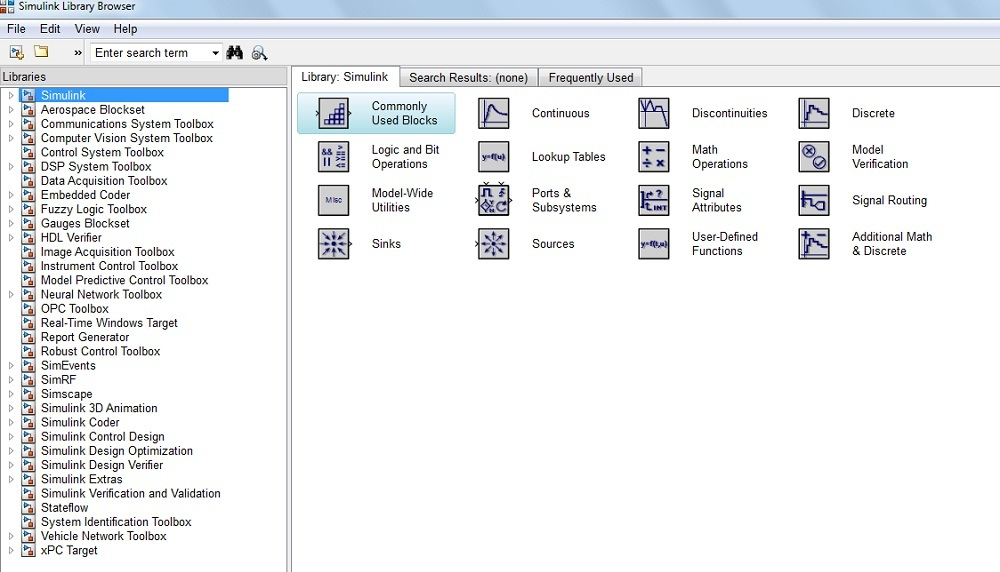# Different Types of MATLAB Simulink Toolbox with Uses

#### Introduction:

MATLAB Simulink is developed by the MathWorks.

What is the Purpose and Use of MATLAB Simulink?

The Simulink library browser contains the collection of multiple tools and their functions. It is useful for the simulation of the dynamic system in the MATLAB environment.

The Simulink toolboxes provide the specific tools for analyzing, designing, simulation of the system, making the communication between the other system, etc.

And also, these toolboxes are required for building the system, designing automatic control systems,  an image designing system, data designing system, algorithms, etc.

The MATLAB toolbox contains multiple functional tools as per your requirements for building dynamic systems or projects.

#### What are the different MATLAB Simulink Toolbox?

Here, we are interested in knowing different Simulink toolbox with their tool functions.

The below diagram shows the list of Simulink toolboxes from MATLAB R2013a software.Note: More or less you will find similar Simulink toolboxes in different versions of MATLAB.

From Mathworks, the list of professional toolboxes is given below.

• Control System Toolbox
• DSP Communication Toolbox
• Fuzzy Logic Toolbox
• OPC Toolbox
• Data Acquisition Toolbox
• Image Acquisition Toolbox
• Robust Control Toolbox
• Vehicle Network Toolbox
• Neural Network Toolbox
• Instrument Control Toolbox
• System Identification Toolbox
• Communication System Toolbox
• Computer Vision System Toolbox

These are top 13 toolboxes include in Simulink libraries browser of MATLAB R2013a software.

These are all the standard Simulink Toolboxes. If you are interested in mastering MATLAB simulation, you should get your hands using and practicing these toolboxes.

#### What are the Applications/Uses of Simulink Toolbox?

Check this list to understand the purpose of different Simulink toolboxes.

• The communication toolbox is useful in industries like telecommunications system, telephonic system, aerospace system, and computer peripherals system.
• The signal processing system toolbox is useful for audio and video image processing system. For example, the scanning machine, digital tape system, fax machine, etc.
• The control system toolbox is used to designing automatic control systems. For example, Washing machine and Camera.
• The Fuzzy Logic Toolbox provides the tools for designing, simulating and analyzing fuzzy inference systems.
• The math function toolbox used for the symbolic computation, variable arithmetic functions.

The list goes on. I have addressed some of the most useful Simulink toolboxes.

What’s Next?

Before this tutorial, I have shared the tutorials base on ‘How to Start and Run the MATLAB Simulink model? [with Example]’.

Keep Learning and Exploring different MATLAB Simulink toolboxes. If you want to share a brief about any Simulink toolbox you are using, please write in the comment section.

Practice Now »

### 12 thoughts on “Different Types of MATLAB Simulink Toolbox with Uses”

1.Thanks for sharing. It’s really helpful and good.

•Thank you for appreciating me.

•Hi,
Thanks for the information you shared.
May i get some PDF books?

•Sorry, I don’t have any other related stuff.

2.great work

•Thank you 🙂

3.What are the online resources to learn specific toolboxes in details?

•Sorry, I am not sure. But I think that, you will get details explanations from offline resources like any standard MATLAB/Simulink books.

4.Nice work Dipali. Thanks

•Thanks, Satyaki 🙂

5.•